Search IntMath
Close

450+ Math Lessons written by Math Professors and Teachers

5 Million+ Students Helped Each Year

1200+ Articles Written by Math Educators and Enthusiasts

Simplifying and Teaching Math for Over 23 Years

# Today is “e” day (Feb 7th)

By Murray Bourne, 07 Feb 2010

"e" is one of those amazing numbers that arises naturally in the scheme of things.

Today, 2/7, is celebrated as "e" Day, since the first 2 digits in the number "e" are 2 and 7.

Other special numbers include "pi" (π = 3.141592653..., celebrated on Mar 14th), which is the circumference of any circle divided by its diameter; and "phi" φ = 1.6180339887..., which is the so-called "beauty ratio". These numbers are irrational (that is, their decimals go on forever and never repeat).

e is also an irrational number and it has value:

e = 2.718281828459...

The number e was "discovered" by several mathematicians (Oughtred, Huygens, Jacob Bernoulli, Mercator and Leibniz) but they didn't quite know they had stumbled on it and didn't necessarily know its significance at first.

There are some curious properties of e, one of which is that it's the limiting value of this expression: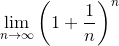## What does this mean?

Let's substitute some values to see what this limit expression means.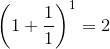Next, we substitute n = 2: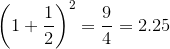One more: n = 3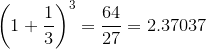It's easier to see what's going on in a graph.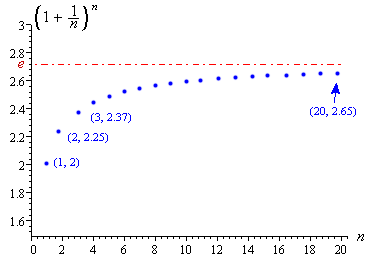I've plotted the values after substituting n = 1 up to n = 20. We can see it's tending (getting closer to) some value. That value, marked as "e" in red on the graph, is 2.718281828459...

This function converges slowly. If I substitute in n = 1000, I only get 2 decimal place accuracy, and even if I go up to n = 100,000, I get 2.718268, which is only accurate to 4 decimal places.

## A better way to find e

The value of "e" can also be found by adding the infinite sum: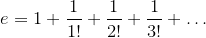The "factorial" exclamation mark, "!", means "multiply by all positive integers smaller than the number given". So

2! = 2 × 1 = 2

and

3! = 3 × 2 × 1 = 6.

We can write our infinite sum using summation notation as: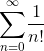I'll show the first few terms of this sum. When n = 0, using the convention 0! = 1, we have: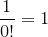When n = 1: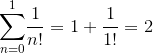When n = 2: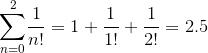And one more, when n = 3: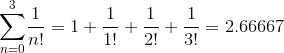This second infinite sum is a more efficient way of finding "e", since we only need to add 9 terms and we have 6 decimal place accuracy.

## Why is "e" so important?

So what is e good for? See Exponential and Logarithmic Functions.

It is used extensively in logarithms (which was the only way to do difficult calculations for hundreds of years before calculators came along), exponential growth (of populations, money or drug concentrations over time) and complex numbers (which were used to design the computer or mobile device you are reading this on).

So happy "e" day (February 7th, or 2/7).

### 10 Comments on “Today is “e” day (Feb 7th)”

1. Head’s Up for e-Day « Let's Play Math! says:

[...] Today is “e” day [...]

2. math lover says:

Pi-day is on 3-14, but Some people consider 22/7 as pi-day.

3. Mike Thayer says:

In analogy with pi day (March 14th) "keeping 2 decimal places", shouldn't e day be on February 71st (or 72nd, if rounding)? 🙂 That's when my precalculus class celebrated it last year - counting forward, I believe that's some time around April 11th.

It'd be easier to do this in a non-"middle-endian" calendrical system: 27/1 (or again, 27/2 if rounding....). Actually, now that I think about it, in a "middle-endian" calendar, pi day is obvious, but e day is not (3/14 vs 2/71), while in "little-endian" calendars, pi day is nonobvious (31/4) but e day is (27/1).....

4. Murray says:

@Mike: Good to hear from you! Thanks for your input. But somehow, April 11th seems rather distant.

How about the idea of celebrating at 4:28 AM on the 7th February (based on 0.01828... × 24 hrs = 4 hrs 23 min). Then again, at that hour, it would be difficult to find anyone who cares! 🙂

5. angeli says:

happy e day
"thanks for this beautifull post.
I loved it"

6. February 7 – “e” day « 365 reasons to celebrate says:

[...] But for those who have the background to understand,  “It is the limit of (1 + 1/n)n as n approaches infinity (source: the almighty wikipedia). It is this [...]

7. Geeking out on the (Math) Holidays | coaches corner blog says:

[…] sure there are other dates out there that should and could be celebrated (i.e. e Day).  So don’t feel too bad if you’re not taking a day this week to graph a Gingerbread […]

8. February 7th – Ballet Day | Ernie's Blog says:

[…] “e” Day  – (Strictly for math geeks). […]

9. Daily Announcements | Science Focus Program says:

[…] Today is “e” Day!   […]

10. Tag der eulerschen Zahl - e-Day - 27. Januar says:

[…] Murray Bourne: Today is “e” day (Feb 7th) – auf: intmath.com am 7. Februar 2010 (englisch) […]

### Comment Preview

HTML: You can use simple tags like <b>, <a href="...">, etc.

To enter math, you can can either:

1. Use simple calculator-like input in the following format (surround your math in backticks, or qq on tablet or phone):
a^2 = sqrt(b^2 + c^2)
(See more on ASCIIMath syntax); or
2. Use simple LaTeX in the following format. Surround your math with $$ and $$.
$$\int g dx = \sqrt{\frac{a}{b}}$$
(This is standard simple LaTeX.)

NOTE: You can mix both types of math entry in your comment.